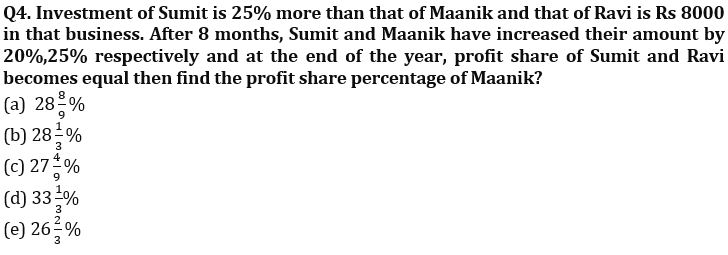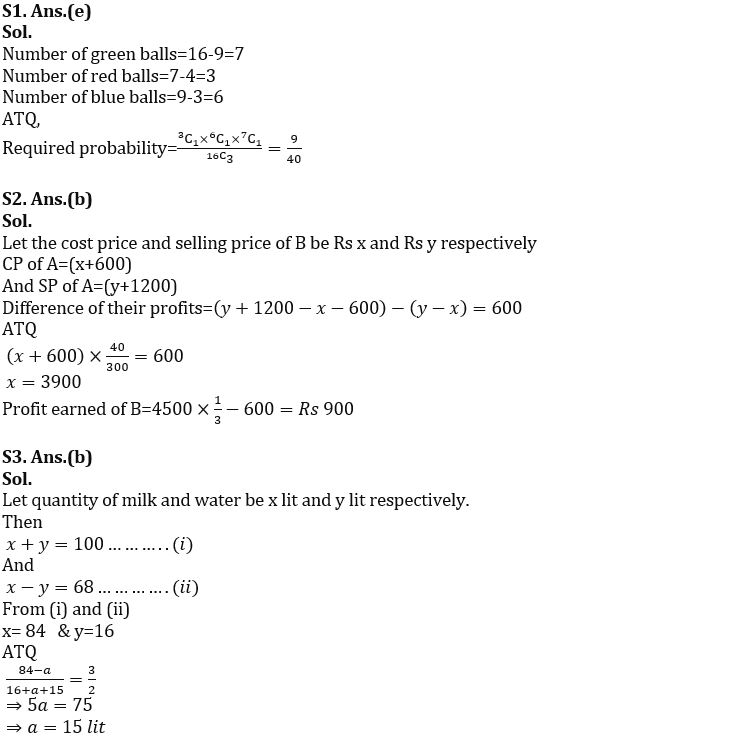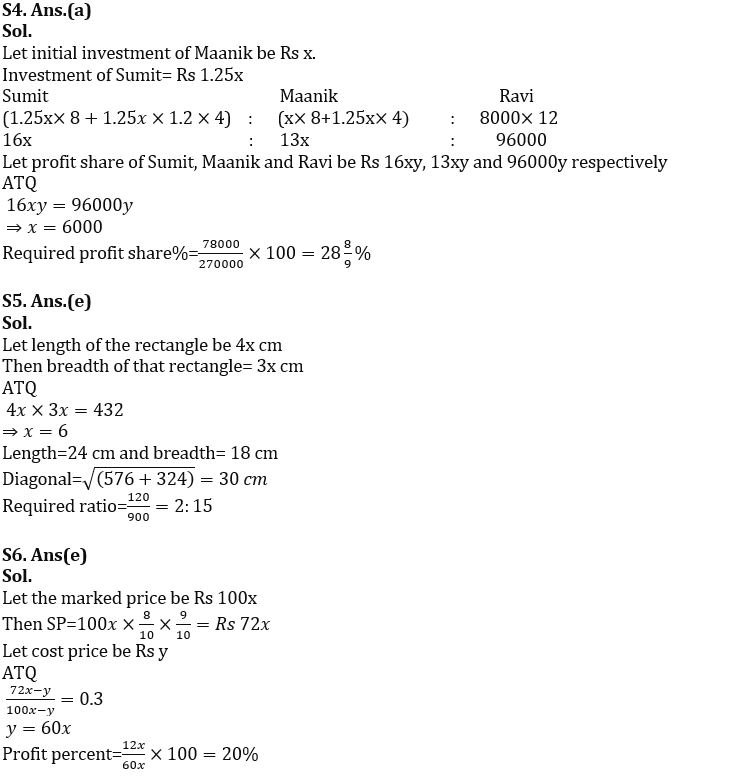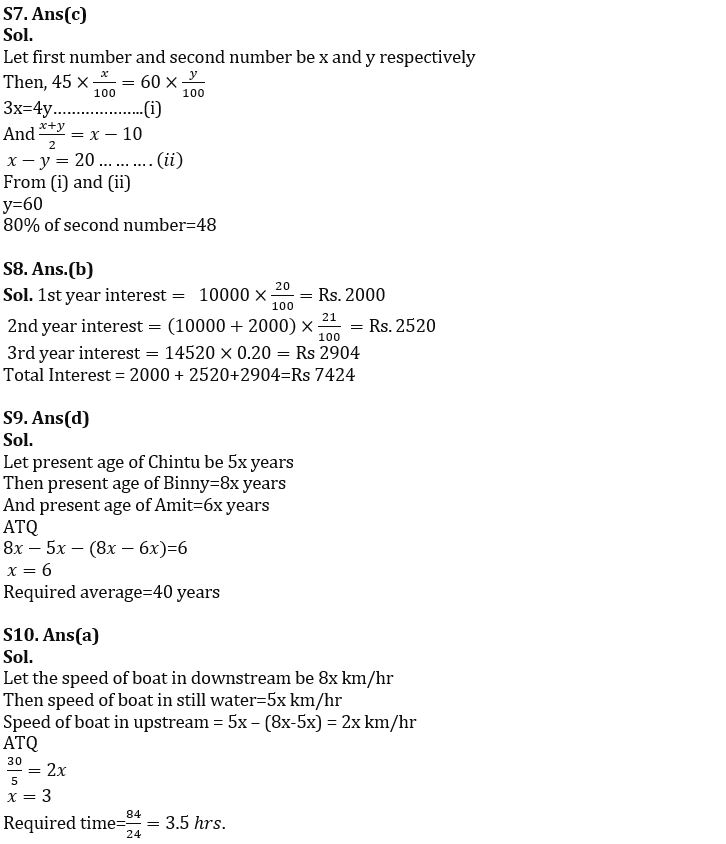Latest Banking jobs   »   sbi po quant

# Quantitative Aptitude Quiz For SBI PO Prelims 2023 -16th September

Q1. In a bag, there are 16 balls of three different colors i.e. red, blue and green. Number of red and blue balls is 9 and difference between red and green ball is 4 then find the probability of getting a ball of each color if three balls are picked at random?
(a) 5/28
(b) 4/27
(c) 7/36
(d) 8/35
(e) 9/40

Q2. Cost price of article A is Rs 600 more than that of B and selling price of A is Rs 1200 more than that of B. If difference between profit earned on selling these two articles is 13⅓% of the cost price of A then find profit earned on B if profit % earned on A is 33⅓%?
(a) Rs 800
(b) Rs 900
(c) Rs 840
(d) Rs 960
(e) Rs 1000

Q3. In 100 lit mixture of milk and water, milk is 68 lit more than that of water. When ‘a’ lit of milk is taken out and (a+15) lit of water is added, milk becomes 50% more than that of water. Find value of ‘a’?
(a) 20
(b) 15
(c) 18
(d) 12
(e) 16Q5. Area of rectangle having length and breadth in the ratio of 4: 3 is 432 cm2. A square whose side is equal to diagonal of rectangle then find ratio of numerical value of perimeter to the area of square?
(a) 7:55
(b) 4:35
(c) None of these
(d) 1:8
(e) 2:15

Q6. A shopkeeper sells a product after allowing two successive discounts of 10% and 20% on it. Find the profit percent if the profit is 30% of the price by which the product is marked up?
(a) 30%
(b) 17.5%
(c) 25%
(d) 15%
(e) 20%

Q7. 45% of first number is equal to 60% of second number. If average of both the numbers is 10 less than the first number, then find 80% of second number?
(a) 64
(b) 40
(c) 48
(d) 56
(e) 80

Q8. Arun invested Rs. 10,000 for three years at CI at the rate of 20% per annum. If in 1st and 3rd year interest is calculated annually and in 2nd year it was calculated half-yearly, then find the total interest received by Arun in three years?
(a) Rs 7554
(b) Rs 7424
(c) Rs 7868
(d) Rs 7262
(e) Rs 7343

Q9. Amit’s present age is 75% of Binny’s present age where as present age of Chintu is 5/8 th of Binny’s present age. If difference between difference of Chintu and Binny age and difference of Binny and Amit age is 6 years then find the average of their age two years later?
(a) 44 years
(b) 42 years
(c) 36 years
(d) 40 years
(e) 38 years

Q10. Speed of boat in still water is 37.5% less than the speed of the boat in downstream and boat covers 30 km in upstream in 5 hours, then find time taken by boat to cover 84 km in downstream?
(a) 3.5 hr
(b) 3 hr
(c) 4.5 hr
(d) 4 hr
(e) 5 hr

Solutions## FAQs

### What are the sections asked in the SBI PO Prelims Exam?

SBI PO Prelims exam consists of Reasoning Ability, Quantitative Reasoning and English Language

#### Congratulations!Union Budget 2023-24: Free PDF